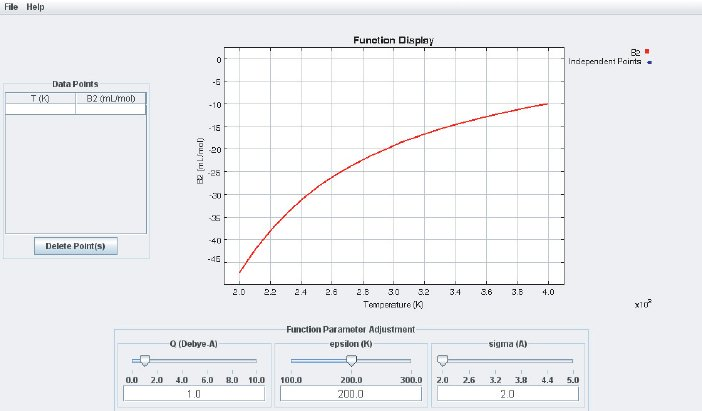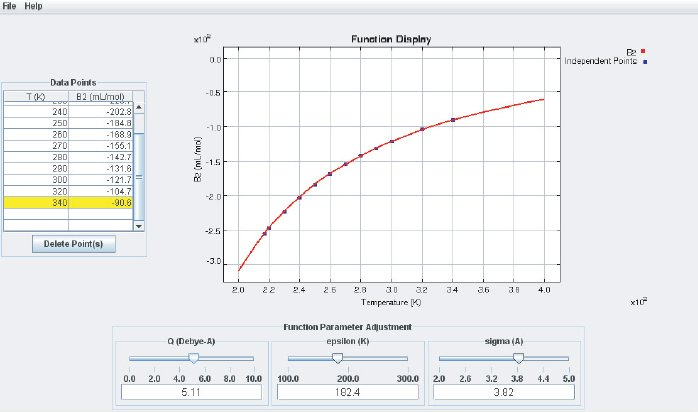The left side of the display is used for numerical data input (B at different temperatures). At the bottom are three sliders and input fields which are used to adjust the basic parameters of the LJ potential ($\varepsilon$ (“epsilon”) and $\sigma$ (“sigma”) according to Eq. (4)) and the quadrupole moment Q. At the center of the interface is the Function Display where the analytical B(T ) calculated from Eq. (7) and additional quadrupole terms is displayed together with the data points. Data points that are entered in the data input field are automatically added as points in the Function Display. If any of the sliders is moved the function B(T) will change accordingly. By adjusting the potential parameters one can bring the calculated B(T) curve to agree with the experimental data, as shown in the screenshot below:This way, the LJ parameters and the quadrupole moment can be obtained from experimentally determined virial coefficients B. These potential parameters can be used for subsequent Molecular Dynamics (MD) or Monte Carlo (MC) simulations. The unit for $\sigma$ is $\text{\AA} = 10^{-10}m$. The parameter $\varepsilon$ corresponds to the depth of the LJ potential well and therefore measures the interaction energy at the molecular level. The usual energy unit in SI is Joule (J) but the value for the interaction between two molecules is tiny compared to a Joule. The Boltzmann constant k is of “atomic dimension”; therefore $\varepsilon$ is often given in units of kT and one simply specifies a temperature T instead. Therefore, the module reads the $\varepsilon$ energy value in Kelvin. The dimension of the quadrupole moment is $charge \cdot length^2$. Again, SI units are adapted to macroscopic objects and lead to very small numerical values at the molecular scale. Here, the quadrupole moment is given in units of $debye \cdot \text{\AA}$ with 1 debye = $1 \cdot 10^{-18}$ esu·cm. The unit esu is a unit of electrical charge and comes from the old CGS unit system (Centimeter–Gram–Second). The corresponding SI unit is $C \cdot m^2$.